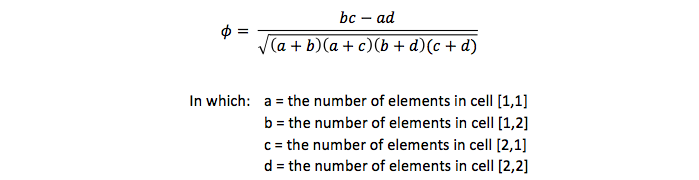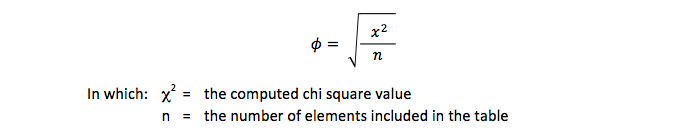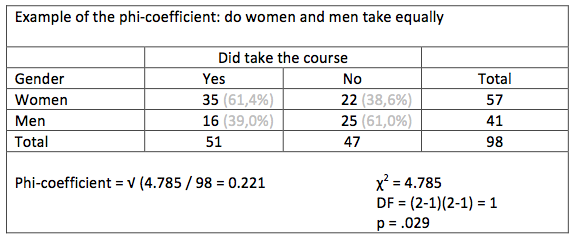Phi-coefficient# If the road is not yet clear to you,we will show you the way how to get through.## The formula of the phi-coefficient

There are two formulas for the phi-coefficient:andAlthough both formulas look very differently computations with the same data give the same outcome.

The second formula is similar to the formula of Cramérs V. Therefore the phi-coefficient can be seen as a special case of Cramérs V.

## How to interpret the phi-coefficient?

The outcome of this formula is always between 0 and 1. The value 0 means there is no cohesion between the two variables. The value 1 means a perfect correspondence: only the cells on the diagonal are filled.

Because we are dealing with variables measured at a nominal level, the rows or columns can be reversed without any problem. Due to this reversibility it is not important if the cells in the diagonal are filled from left above to right down or from left down to right above. The sign (that is minus (-) or plus (+)) is not important.

## Testing the strenght of the phi-coefficient

The phi-coefficient is tested with the chi square test. This is a little bit odd, because the chi square value is also part of the formula for computing the phi-coefficient too.

## An example of computing the phi-coefficient

One might wonder if women more often take a course on self-defence than man. Now two variables are needed: gender (woman = 1, man = 2) and did take the course (no = 0, yes = 1). For 98 youngsters between 15 and 30, these numbers were found:Without the percentages the differences are not easily seen. It really helps to interpret the result.

Use our SPSS tutorials to learn how to run this test in SPSS.

## Mission

My goal is to teach you how to conduct good research.

Because:

Good research provides you with better information.
With better information, you can make better decisions.
With better decisions, you can create a healthier, wealthier and freer world,
for people, fauna and flora, for current and future generations.

That is why I think it is important that  you know how to do your research well.